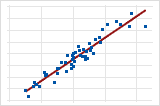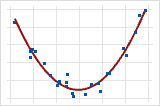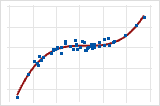# What is model order?

Model order is the type of model used to show a trend in the data. The model order is an important factor in how accurately the model describes the data and predicts a response.

For example, a linear model can show a steady rate of increase or decrease in the data. A quadratic model (often approximately in the shape of a U or an inverted U) can explain curvature in the data. A cubic model can describe a "peak-and-valley" pattern in the data.

Model order Example

Linear

Y = bo + b1X

(first order)Y = bo + b1X + b11X2

(second order)Cubic

Y = bo + b1X + b11X2 + b111 X3

(third order)Each model order corresponds with the degree of the equation (the highest power of the X variable) used to generate the model, where Y is the response, X is the predictor, bo is the intercept, and b1, b11, and b111 are the coefficients.

By using this site you agree to the use of cookies for analytics and personalized content.  Read our policy